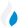•Trade Pi
•Trade
•Exchange
•Trade Pi
•Trade
•Exchange
\$1.13T
Total marketcap
\$51.79B
Total volume
40.02%
BTC dominance
•Trade Pi
•Trade
•Exchange

# ALICE to ETH Exchange Rate - 1 My Neighbor Alice in Ethereum

0.0011403036938791
• BTC 0.00008
• ETH 0.0011
Vol [24h]
\$21,201.71

## alice to eth converter

Exchange Pair Price 24h volume
KuCoin ALICE/ETH \$1.91 \$17.99K
Gate.io ALICE/ETH \$1.88 \$3.16K
Uniswap ALICE/ETH \$0 \$0
BTSE ALICE/ETH \$0 \$0

## ALICE/ETH Exchange Rate Overview

Name Ticker Price % 24h 24h high 24h low 24h volume
My Neighbor Alice alice \$1.89 1.2044% \$1.9 \$1.83 \$21.67M
Ethereum eth \$1,680.46 0.8928% \$1,690.92 \$1,649.82 \$6.58B

Selling 1 ALICE you get 0.0011403036938791 Ethereum eth.

My Neighbor Alice Mar 15, 2021 had the highest price, at that time trading at its all-time high of \$40.93.

692 days have passed since then, and now the price is 4.61% of the maximum.

Based on the table data, the ALICE vs ETH exchange volume is \$21,201.71.

Using the calculator/converter on this page, you can make the necessary calculations with a pair of My Neighbor Alice/Ethereum.

## Q&A

### What is the current ALICE to ETH exchange rate?

Right now, the ALICE/ETH exchange rate is 0.0011403036938791.

### What has been the My Neighbor Alice to Ethereum trading volume in the last 24 hours?

Relying on the table data, the My Neighbor Alice to Ethereum exchange volume is \$21,201.71.

### How can I calculate the amount of ETH? / How do I convert my My Neighbor Alice to Ethereum?

You can calculate/convert ETH from My Neighbor Alice to Ethereum converter. Also, you can select other currencies from the drop-down list.

## ALICE to ETH Сonversion Table

ALICE ETH
0.2 ALICE = 0.0002 ETH
0.5 ALICE = 0.0005 ETH
1 ALICE = 0.001 ETH
2 ALICE = 0.002 ETH
3 ALICE = 0.003 ETH
5 ALICE = 0.005 ETH
8 ALICE = 0.009 ETH
30 ALICE = 0.03 ETH
60 ALICE = 0.06 ETH
600 ALICE = 0.6 ETH
6000 ALICE = 6.8 ETH
60000 ALICE = 68.4 ETH
600000 ALICE = 684.1 ETH